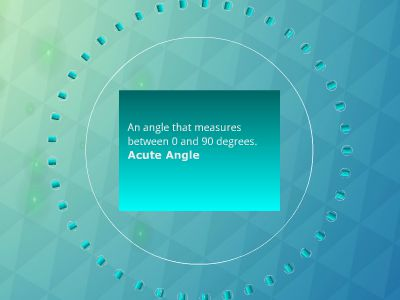Publications: 31 | Followers: 0

## GEOMETRY - cdn.compknowhow.com

Publish on Category: Birds 0

An angle that measures between 0 and 90 degrees.Acute Angle
To cut in half.Bisect
The point where the angle bisectors of a triangle meet.Incenter
A polygon that is both equilateral and equiangular.Regular Polygon
Objects that lie in the same plane.Coplanar
Two angles whose measures add up to ninety degrees.Complementary Angles
Two adjacent angles whose noncommon sides form opposite rays.Linear Pair
A segment that connects the midpoints of two sides of a triangle.Midsegment
A segment that connects a vertex of a triangle with the midpoint of the opposite side.Median
A triangle with three congruent sides.Equilateral/Equiangular/Acute/Regular/Isosceles
The distance around a figure.Perimeter
A polygon with four congruent sides.Equilateral Quadrilateral
Objects that have the same angle measures and the same side lengths.Congruent Figures
Every point on the ______ is equidistant from the endpoints of the segment.Perpendicular Bisector
The side of a right triangle opposite the right angle.Hypotenuse
A point that divides a segment into two congruent segments.Midpoint
c2= a2+ b2.The Pythagorean Theorem
Two angles whose measures add up to one hundred and eighty degrees.Supplementary Angles
The angles adjacent to the base.Base Angles
The angle in an isosceles triangle opposite the base.Vertex Angle
Lines that intersect to form a right angle.Perpendicular Lines
Points that lie on the same line.Collinear Points
A non-rigid transformation.Dilation
An educated guess based on observations.Conjecture
The ratio of rise over run between two points on a coordinate plane.Slope
The point of concurrency of the medians of a triangle.Centroid
What you need to prove a conjecture is false.Counterexample
A statement that is accepted without proof.Postulate
A polygon that has no “dents”.Convex Polygon
The point of concurrency of the altitudes in a triangle.Orthocenter
The point of intersection of the perpendicular bisectors of a triangle.Circumcenter
A polygon that has a dent.Concave
A series of statements and reasons that explain why something is true.Proof
A triangle with at least two congruent sides.Isosceles Triangle
The words that follow “then” in a conditional statement.Conclusion
A line that intersects two other lines at different points.Transversal
A segment or line that goes from a vertex of a triangle to the opposite side or to a line that contains the opposite side and makes a right angle.Altitude
Lines that do not intersect and are not coplanar.Skew Lines
The words that follow “if” in a conditional statement.Hypothesis
The two sides of a right triangle that form the right angle.Legs of a right triangle
A statement that contains the phrase “if and only if.”Biconditional statement
Coplanar lines that do not intersect.Parallel Lines

0

Embed

Share